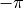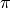# control.bode_plot¶

control.bode_plot(syslist, omega=None, plot=True, omega_limits=None, omega_num=None, margins=None, method='best', *args, **kwargs)[source]

Bode plot for a system

Plots a Bode plot for the system over a (optional) frequency range.

Parameters
• syslist (linsys) – List of linear input/output systems (single system is OK)

• omega (array_like) – List of frequencies in rad/sec to be used for frequency response

• dB (bool) – If True, plot result in dB. Default is false.

• Hz (bool) – If True, plot frequency in Hz (omega must be provided in rad/sec). Default value (False) set by config.defaults[‘freqplot.Hz’]

• deg (bool) – If True, plot phase in degrees (else radians). Default value (True) config.defaults[‘freqplot.deg’]

• plot (bool) – If True (default), plot magnitude and phase

• omega_limits (array_like of two values) – Limits of the to generate frequency vector. If Hz=True the limits are in Hz otherwise in rad/s.

• omega_num (int) – Number of samples to plot. Defaults to config.defaults[‘freqplot.number_of_samples’].

• margins (bool) – If True, plot gain and phase margin.

• method (method to use in computing margins (see stability_margins())) –

• *args (matplotlib.pyplot.plot() positional properties, optional) – Additional arguments for matplotlib plots (color, linestyle, etc)

• **kwargs (matplotlib.pyplot.plot() keyword properties, optional) – Additional keywords (passed to matplotlib)

• grid (bool) – If True, plot grid lines on gain and phase plots. Default is set by config.defaults[‘freqplot.grid’].

• initial_phase (float) – Set the reference phase to use for the lowest frequency. If set, the initial phase of the Bode plot will be set to the value closest to the value specified. Units are in either degrees or radians, depending on the deg parameter. Default is -180 if wrap_phase is False, 0 if wrap_phase is True.

• wrap_phase (bool or float) – If wrap_phase is False (default), then the phase will be unwrapped so that it is continuously increasing or decreasing. If wrap_phase is True the phase will be restricted to the range [-180, 180) (or [,) radians). If wrap_phase is specified as a float, the phase will be offset by 360 degrees if it falls below the specified value. Default value is False and can be set using config.defaults[‘freqplot.wrap_phase’].

• reset (The default values for Bode plot configuration parameters can be) –

• dictionary (using the config.defaults) –

• 'bode'. (with module name) –

Returns

• mag (ndarray (or list of ndarray if len(syslist) > 1))) – magnitude

• phase (ndarray (or list of ndarray if len(syslist) > 1))) – phase in radians

• omega (ndarray (or list of ndarray if len(syslist) > 1))) – frequency in rad/sec

Notes

1. Alternatively, you may use the lower-level methods LTI.frequency_response() or sys(s) or sys(z) or to generate the frequency response for a single system.

2. If a discrete time model is given, the frequency response is plotted along the upper branch of the unit circle, using the mapping z = exp(1j * omega * dt) where omega ranges from 0 to pi/dt and dt is the discrete timebase. If timebase not specified (dt=True), dt is set to 1.

Examples

>>> G = ct.ss([[-1, -2], [3, -4]], [, ], [[6, 8]], [])
>>> Gmag, Gphase, Gomega = ct.bode_plot(G)